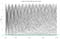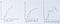Tales about data, statistics, machine learning, visualisation, and much more. By Adrià Luz (@adrialuz) and Sara Gaspar (@sargaspar).

# On the role of dummy variables and interactions in linear regression

## Understanding this will help you be more in control when fitting linear models

We’re all familiar with the quintessential example of linear regression: predicting house prices based on house size, number of rooms and bathrooms, and so on. However, we may often want to introduce categorical variables into our model too, such as whether the house has got a swimming pool or its neighbourhood.

One issue with linear regression models is that they can only interpret numerical inputs. Thus, we need a way of translating words like neighbourhood names to numbers that the model can understand.

The most common way of doing this is by creating dummy variables. Let’s say we’re looking at…

# On A/A tests, p-values, significance, and the uniform distribution

## Simulating 1,000 A/A tests to understand how p-values are distributed

A couple of weeks ago, I was simulating some A/A tests data for my workmate Andrew. We wanted to produce a chart like the below for a company-wide presentation he was doing that week about A/B testing and the dangers of peeking:Image by author

The goal was to show how p-values moved around and how, even with an A/A test where the null hypothesis should definitely be true, we saw p-values < 0.05 at some point.

Hence, I went on to simulate 1,000 A/A tests in order to calculate how many of them reached significance (p-value < 0.05) at some point. Each…

# The bootstrap — or why you should care about uncertainty

## by Adrià Luz

As the Bruce brothers explain in their excellent book Practical Statistics for Data Scientists, one easy and effective way to estimate the sampling distribution of a statistic is to draw additional samples (with replacement) from the sample itself and recalculate the statistic for each resample. This procedure is called the bootstrap.

Resampling is the process of taking repeated samples from observed data (i.e. the dataset), and it includes both the bootstrap and permutation tests. I will cover permutation tests in a future post.

The bootstrap is widely used to find and plot the sampling distribution of a statistic (e.g. mean)…

# Predicting house prices with linear regression

## by Sara Gaspar

Inspired by Kevin Markham’s post on linear regression, Chapter 3 of An Introduction to Statistical Learning and Andrew Ng’s Machine Learning course.

We’ll be working with a dataset of house prices from Kaggle.

## Simple Linear Regression

Simple linear regression is a statistical approach for modelling the relationship between a predictor variable X and a response variable Y. It assumes there is a linear relationship between these two variables and we use that to predict a quantitative output.

Simple linear regression is a very simple approach for supervised learning. But, even though it’s probably the simplest and most straightforward method, it’s the fundamental starting…

# Visualising football players in two dimensions with PCA

## by Adrià Luz

As Trevor Hastie and others explain in the book Introduction to Statistical Learning, when we are faced with a large set of correlated variables, principal components allow us to summarise this set with a smaller number of representative variables that collectively explain most of the variability in the original set. Principal Components Analysis (PCA) is an unsupervised machine learning algorithm that performs dimensionality reduction and allows us to do just that.

To illustrate how PCA works, let’s look at this scatter plot of two variables (x1 and x2). PCA finds the vector u1, whose direction is the one with greater…

# Data Visualisation Mini Series (I): Scatterplots

## by Sara Gaspar

Scatterplots are used to display values from two variables, each variable along one of the axes, allowing us to detect if there is any correlation or potential relationship between them as well as finding if there are any outliers in the data.

Scatterplots are great to pair numerical variables and see if there exists any positive or negative relationship between them. …

# Why you should be plotting learning curves in your next machine learning project

## Spoiler: they will help you understand whether your model suffers from high variance or high bias — and I’ll explain what you can do about itImage by author

The bias-variance dilemma is a widely known problem in the field of machine learning. Its importance is such, that if you don’t get the trade-off right, it won’t matter how many hours or how much money you throw at your model.

In the illustration above, you can get a feel for what bias and variance are as well as how they can affect your model performance. The first chart shows a model (blue line) that is underfitting the training data (red crosses). This model is biased, because it “assumes” the relationship between the independent variable and the dependent variable is…